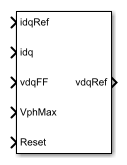# Induction Machine Current Controller

Discrete-time induction machine current PI controller

• Library:
• Simscape / Electrical / Control / Induction Machine Control

•## Description

The Induction Machine Current Controller implements discrete-time proportional-integral (PI) based induction machine current control in the rotor d-q reference frame. You typically use the Induction Machine Current Controller in a series of blocks that make up a control structure. For example, to convert the dq0 reference frame output voltage to voltage in an abc reference frame, connect the Induction Machine Current Controller to an Inverse Clarke Transform in the control structure.

### Equations

The block uses the backward Euler discretization method.

Two PI current controllers that are implemented in the rotor reference frame produce the reference voltage vector:

`${v}_{d}^{ref}=\left({K}_{p_id}+{K}_{i_id}\frac{{T}_{s}z}{z-1}\right)\left({i}_{d}^{ref}-{i}_{d}\right)+{v}_{d_FF},$`

and

`${v}_{q}^{ref}=\left({K}_{p_iq}+{K}_{i_iq}\frac{{T}_{s}z}{z-1}\right)\left({i}_{q}^{ref}-{i}_{q}\right)+{v}_{q_FF},$`

where

• ${v}_{d}^{ref}$, and ${v}_{q}^{ref}$ are the d-axis and q-axis reference voltages, respectively.

• ${i}_{d}^{ref}$, and ${i}_{q}^{ref}$ are the d-axis and q-axis reference currents, respectively.

• ${i}_{d}$ and ${i}_{q}$ are the d-axis and q-axis currents, respectively.

• Kp_id, and Kp_iq are the proportional gains for the d-axis and q-axis controllers, respectively.

• Ki_id and Ki_iq are the integral gains for the d-axis and q-axis controllers, respectively.

• vd_FF, and vq_FF are the feedforward voltages for the d-axis and q-axis, respectively. The feedforward voltages are obtained from the machine mathematical equations and provided as inputs.

• Ts, is the sample time of the discrete controller.

### Voltage Saturation

Saturation is imposed when the stator voltage vector exceeds the voltage phase limit Vph_max:

`$\sqrt{{v}_{d}^{2}+{v}_{q}^{2}}\le {V}_{ph_max},$`

where vd, and vq are the d-axis and q-axis voltages, respectively.

In the case of axis prioritization, the voltages v1 and v2 are introduced, where:

• For d-axis prioritization — v1 = vd and v2 = vq.

• For q-axis prioritizationv1 = vq and v2 = vd.

The constrained (saturated) voltages ${v}_{1}^{sat}$ and ${v}_{2}^{sat}$ are obtained as:

`${v}_{1}^{sat}=\text{min}\left(\mathrm{max}\left({v}_{1}^{unsat},-{V}_{ph_max}\right),{V}_{ph_max}\right)$`

and

`${v}_{2}^{sat}=\text{min}\left(\mathrm{max}\left({v}_{2}^{unsat},-{V}_{2_max}\right),{V}_{2_max}\right),$`

where:

• ${v}_{1}^{unsat}$ and ${v}_{2}^{unsat}$ are the unconstrained (unsaturated) voltages.

• v2_max is the maximum value of v2 that does not exceed the voltage phase limit. The equation that define v2_max is ${v}_{2_max}=\sqrt{{\left({V}_{ph_max}\right)}^{2}-{\left({v}_{1}^{sat}\right)}^{2}}.$

In the case of d-q equivalence, the direct and quadrature axes have the same priority, and the constrained voltages are:

`${v}_{d}^{sat}=\text{min}\left(\mathrm{max}\left({v}_{d}^{unsat},-{V}_{d_max}\right),{V}_{d_max}\right)$`

and

`${v}_{q}^{sat}=\text{min}\left(\mathrm{max}\left({v}_{q}^{unsat},-{V}_{q_max}\right),{V}_{q_max}\right),$`

where:

`${V}_{d_max}=\frac{{V}_{ph_max}|{v}_{d}^{unsat}|}{\sqrt{{\left({v}_{d}^{unsat}\right)}^{2}+{\left({v}_{q}^{unsat}\right)}^{2}}}$`

and

`${V}_{q_max}=\frac{{V}_{ph_max}|{v}_{q}^{unsat}|}{\sqrt{{\left({v}_{d}^{unsat}\right)}^{2}+{\left({v}_{q}^{unsat}\right)}^{2}}}.$`

### Integral Anti-Windup

An anti-windup mechanism is employed to avoid the saturation of the integrator output. In such a situation, the integrator gains become:

`${K}_{i_id}+{K}_{aw_id}\left({v}_{d}^{sat}-{v}_{d}^{unsat}\right)$`

and

`${K}_{i_iq}+{K}_{aw_iq}\left({v}_{q}^{sat}-{v}_{q}^{unsat}\right),$`

where Kaw_id, Kaw_iq, and Kaw_if are the anti-windup gains for the d-axis, q-axis, and field controllers, respectively.

### Assumptions and Limitations

• The plant model for the direct and quadrature axes can be approximated with a first-order system.

## Ports

### Input

expand all

Desired d- and q-axis currents for control of the induction machine, in A.

Data Types: `single` | `double`

Actual d- and q-axis currents of the controlled induction machine, in A.

Data Types: `single` | `double`

Feedforward pre-control voltages, in V.

Data Types: `single` | `double`

Maximum allowable voltage in each phase, in V.

Data Types: `single` | `double`

External reset signal (rising edge) for integrators.

Data Types: `Boolean`

### Output

expand all

Desired d- and q-axis voltages for control of the induction machine, in V.

Data Types: `single` | `double`

## Parameters

expand all

Control Parameters

Proportional gain for direct-axis current control.

Integral gain for direct-axis current control.

Anti-windup gain for direct-axis current control.

Proportional gain for quadrature-axis current control.

Integral gain for quadrature-axis current control.

Anti-windup gain for quadrature-axis current control.

Time, in s, between consecutive block executions. During execution, the block produces outputs and, if appropriate, updates its internal state. For more information, see What Is Sample Time? and Specify Sample Time.

If this block is inside a triggered subsystem, inherit the sample time by setting this parameter to `-1`. If this block is in a continuous variable-step model, specify the sample time explicitly using a positive scalar.

Prioritize or maintain the ratio between the d- and q-axes when the block limits voltage.

Enable or disable pre-control voltage.

## Version History

Introduced in R2017b